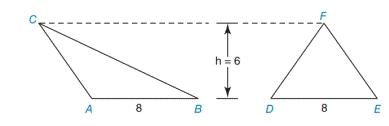Chapter 8.1, Problem 8EElementary Geometry For College St...

7th Edition
Alexander + 2 others
ISBN: 9781337614085

Solutions

Chapter
SectionElementary Geometry For College St...

7th Edition
Alexander + 2 others
ISBN: 9781337614085
Textbook Problem

Are △ A B C and △ D E F congruent?Exercises 7, 8

To determine

ABC and DEF are congruent or not.

Explanation

1) Approach:

Two polygons are congruent if they are the same size and shape,

(i.e.) If their corresponding angles and sides are equal.

2) Calculation:

Given,

The area of the first triangle (ΔABC):

Here, b=8 and h=6

Area of the triangle

A=12bh

=12(8)(6)

=482

A=24 unit2

The area of the second triangle (ΔDEF):

Here, <

Still sussing out bartleby?

Check out a sample textbook solution.

See a sample solution

The Solution to Your Study Problems

Bartleby provides explanations to thousands of textbook problems written by our experts, many with advanced degrees!

Get Started

In Problems 25-38, find y'. 37.

Mathematical Applications for the Management, Life, and Social Sciences

In Exercises 7-28, perform the indicated operations and simplify each expression. 11. 583(3t+2)+13

Applied Calculus for the Managerial, Life, and Social Sciences: A Brief Approach

List the first five terms of the sequence. 7. an=1(n+1)!

Single Variable Calculus: Early Transcendentals